Ex 6.5

Chapter 6 Class 12 Application of Derivatives
Serial order wise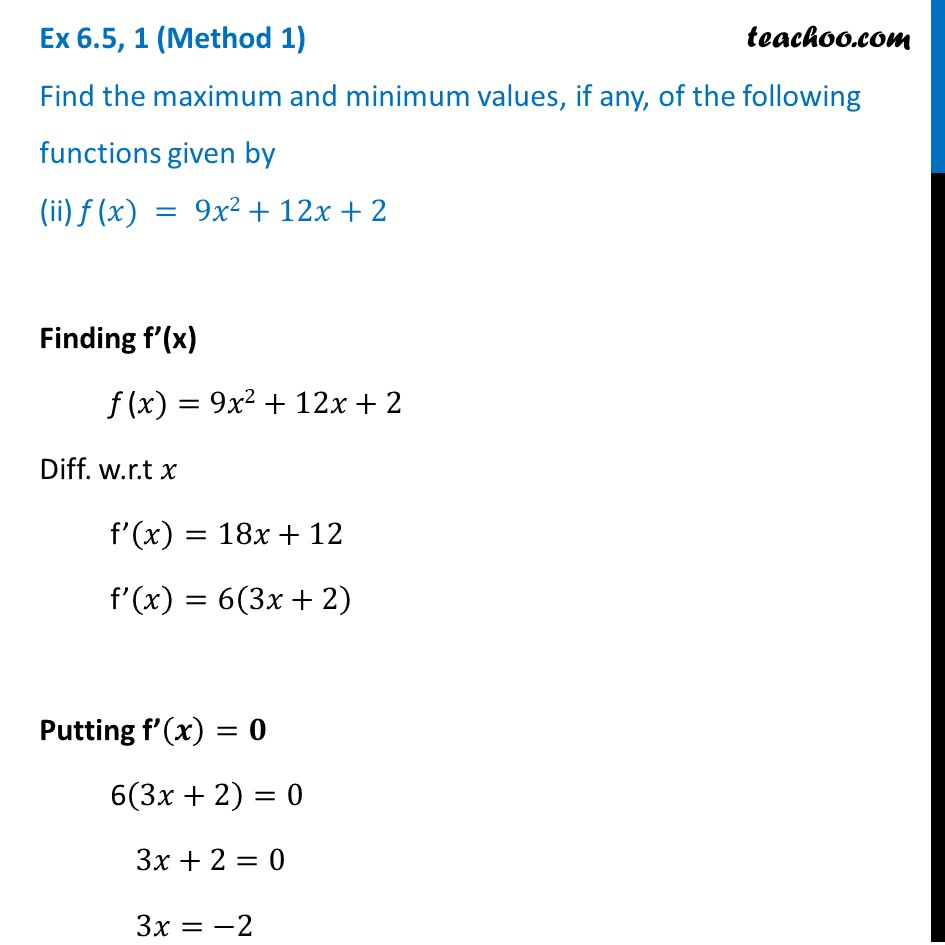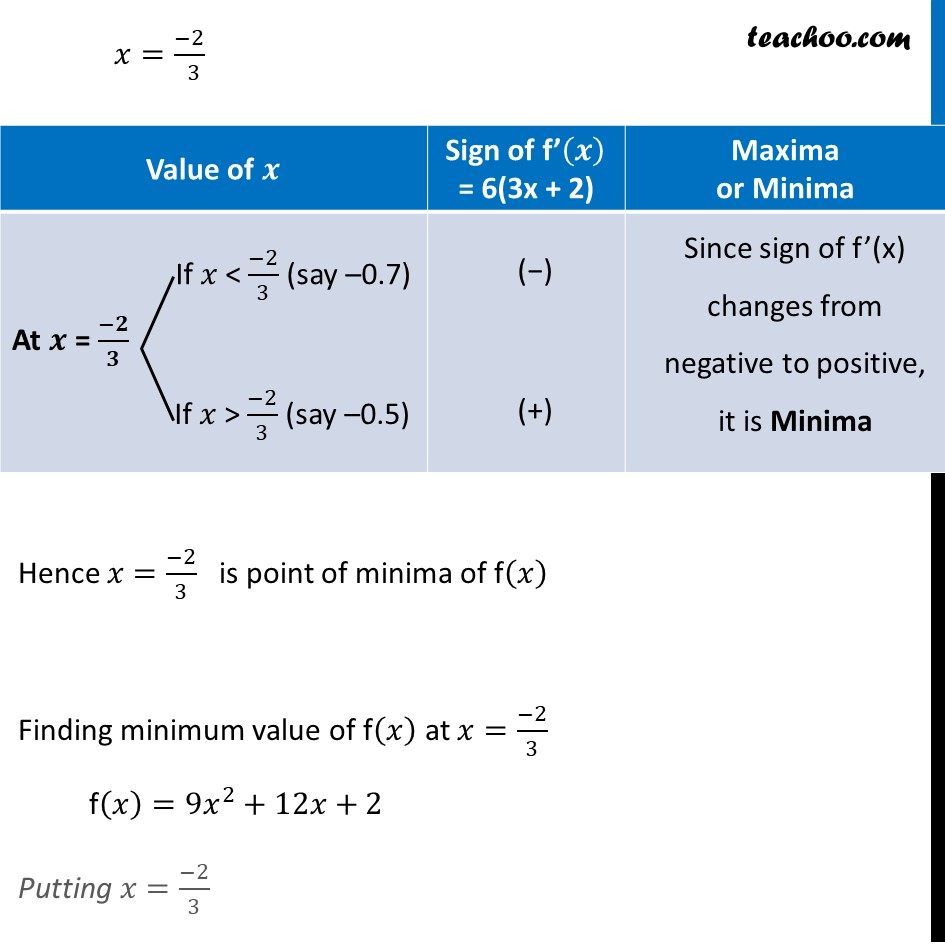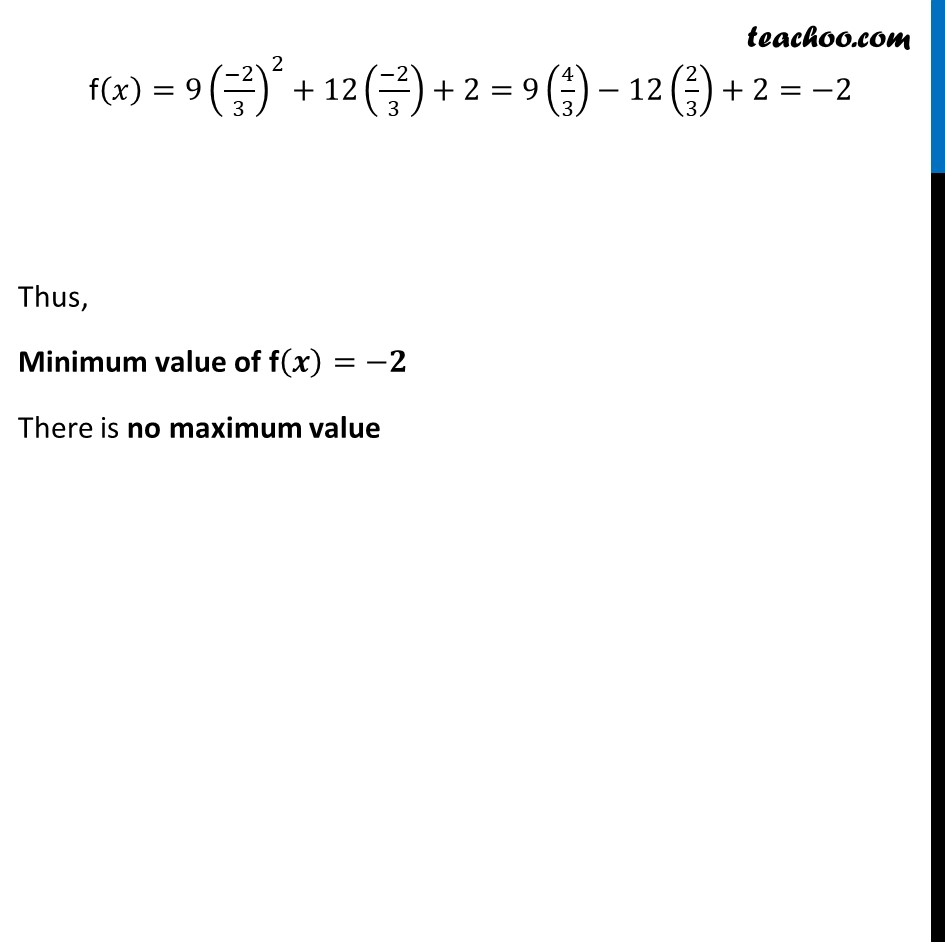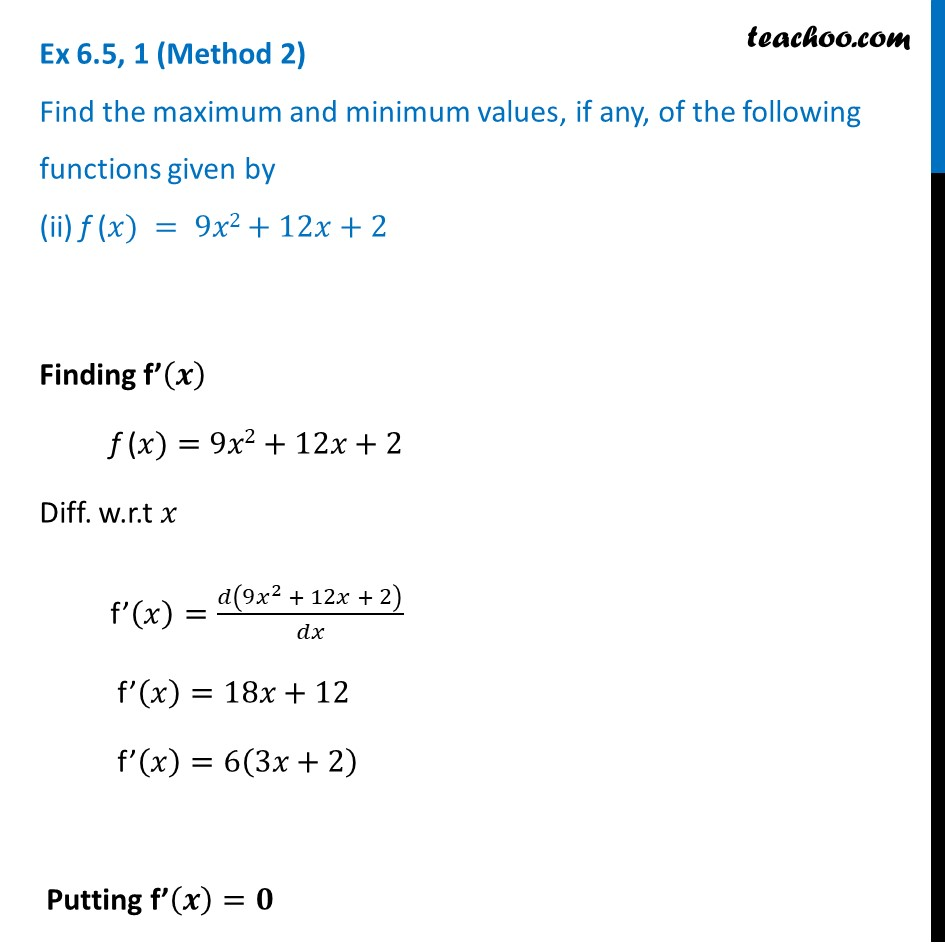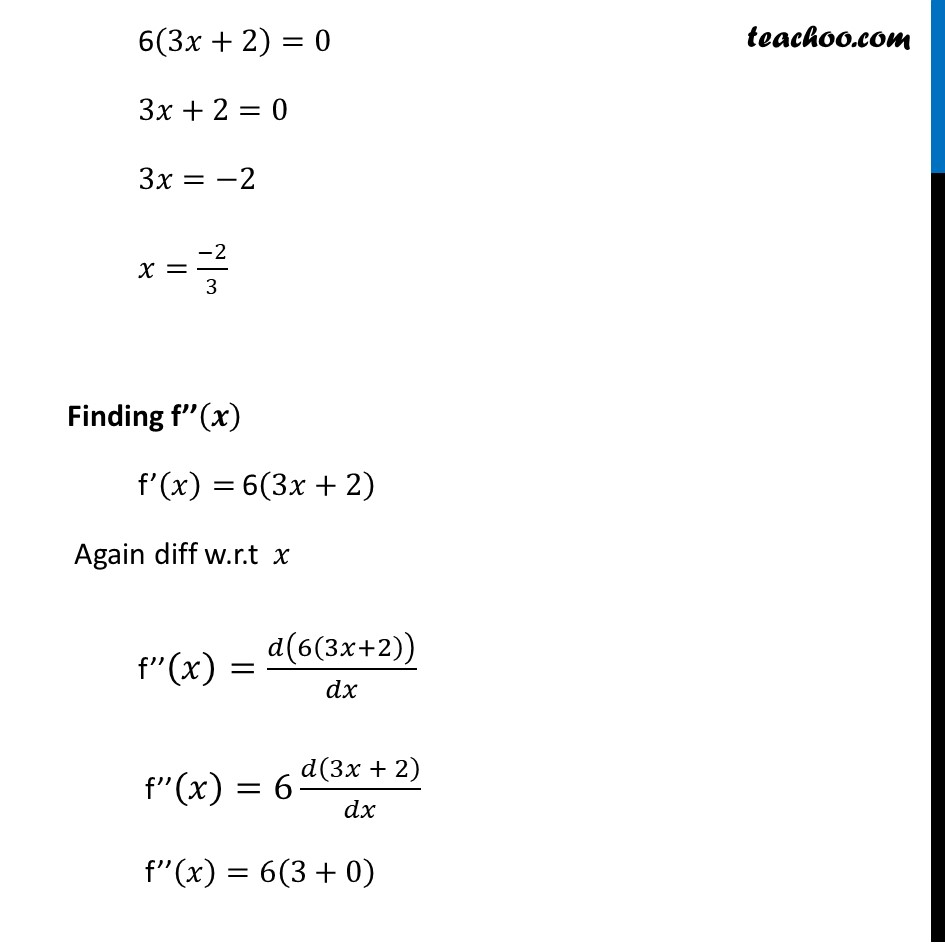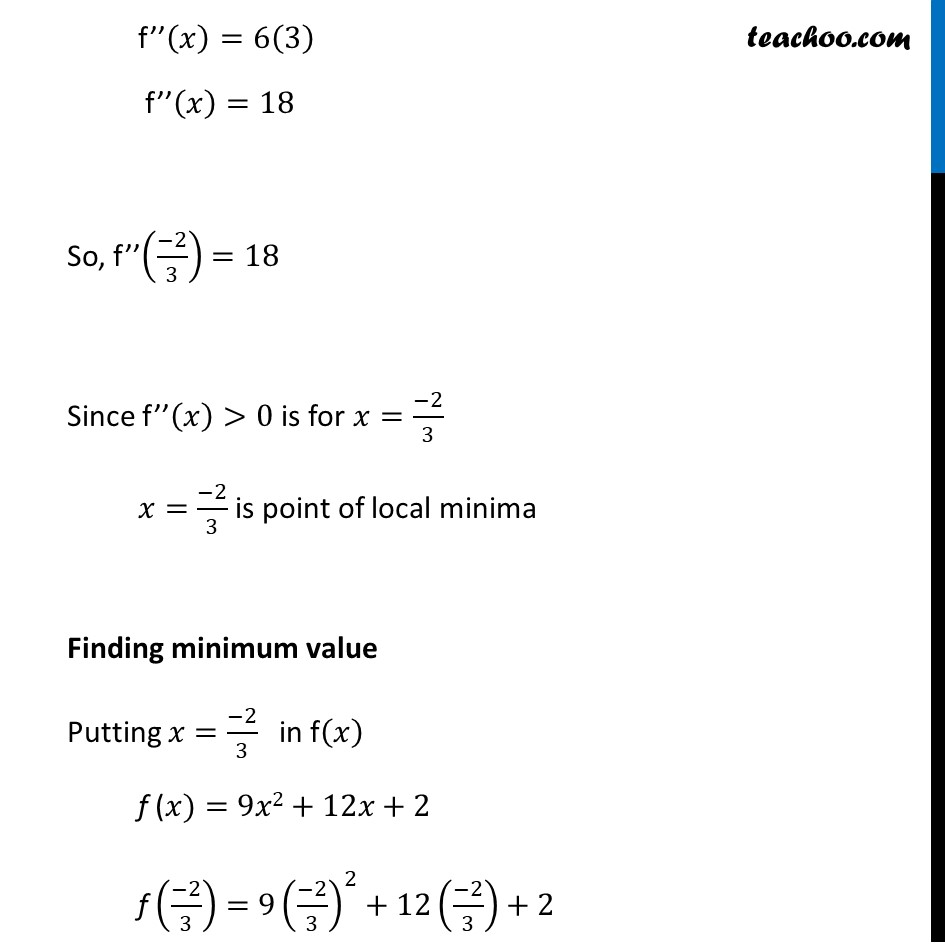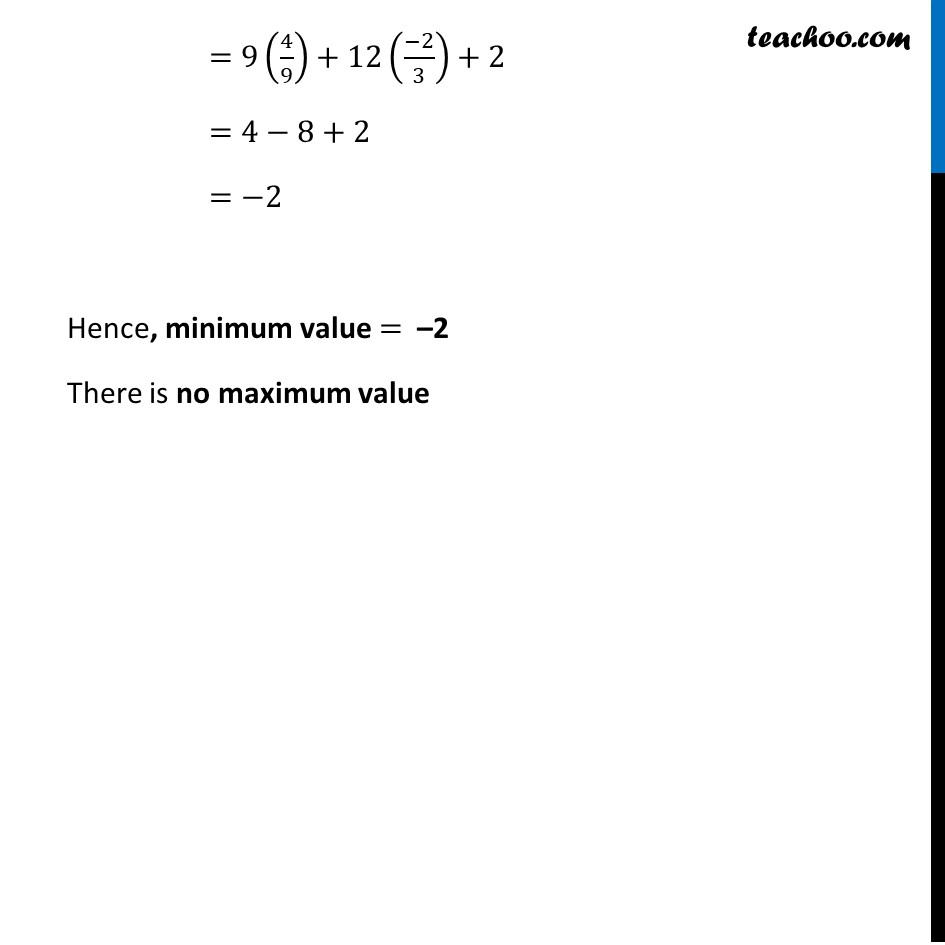Get live Maths 1-on-1 Classs - Class 6 to 12

### Transcript

Ex 6.5, 1 (Method 1) Find the maximum and minimum values, if any, of the following functions given by (ii) f (𝑥) = 9𝑥2+12𝑥+2Finding f’(x) f (𝑥)=9𝑥2+12𝑥+2 Diff. w.r.t 𝑥 f’(𝑥)=18𝑥+12 f’(𝑥)=6(3𝑥+2) Putting f’(𝒙)=𝟎 6(3𝑥+2)=0 3𝑥+2=0 3𝑥=−2 𝑥=(−2)/( 3) Hence 𝑥=(−2)/3 is point of minima of f(𝑥) Finding minimum value of f(𝑥) at 𝑥=(−2)/3 f(𝑥)=9𝑥^2+12𝑥+2 Putting 𝑥=(−2)/3 f(𝑥)=9((−2)/3)^2+12((−2)/3)+2=9(4/3)−12(2/3)+2=−2 Thus, Minimum value of f(𝒙)=−𝟐 There is no maximum value Ex 6.5, 1 (Method 2) Find the maximum and minimum values, if any, of the following functions given by (ii) f (𝑥) = 9𝑥2+12𝑥+2Finding f’(𝒙) f (𝑥)=9𝑥2+12𝑥+2 Diff. w.r.t 𝑥 f’(𝑥)=𝑑(9𝑥^2 + 12𝑥 + 2)/𝑑𝑥 f’(𝑥)=18𝑥+12 f’(𝑥)=6(3𝑥+2) Putting f’(𝒙)=𝟎 6(3𝑥+2)=0 3𝑥+2=0 3𝑥=−2 𝑥=(−2)/3 Finding f’’(𝒙) f’(𝑥)= 6(3𝑥+2) Again diff w.r.t 𝑥 f’’(𝑥)=𝑑(6(3𝑥+2))/𝑑𝑥 f’’(𝑥)=6 𝑑(3𝑥 + 2)/𝑑𝑥 f’’(𝑥)=6(3+0) f’’(𝑥)=6(3) f’’(𝑥)=18 So, f’’((−2)/3)=18 Since f’’(𝑥)>0 is for 𝑥=(−2)/3 𝑥=(−2)/3 is point of local minima Finding minimum value Putting 𝑥=(−2)/3 in f(𝑥) f (𝑥)=9𝑥2+12𝑥+2 f ((−2)/3)=9((−2)/3)^2+12((−2)/3)+2 =9(4/9)+12((−2)/3)+2 =4−8+2 =−2 Hence, minimum value = –2 There is no maximum value